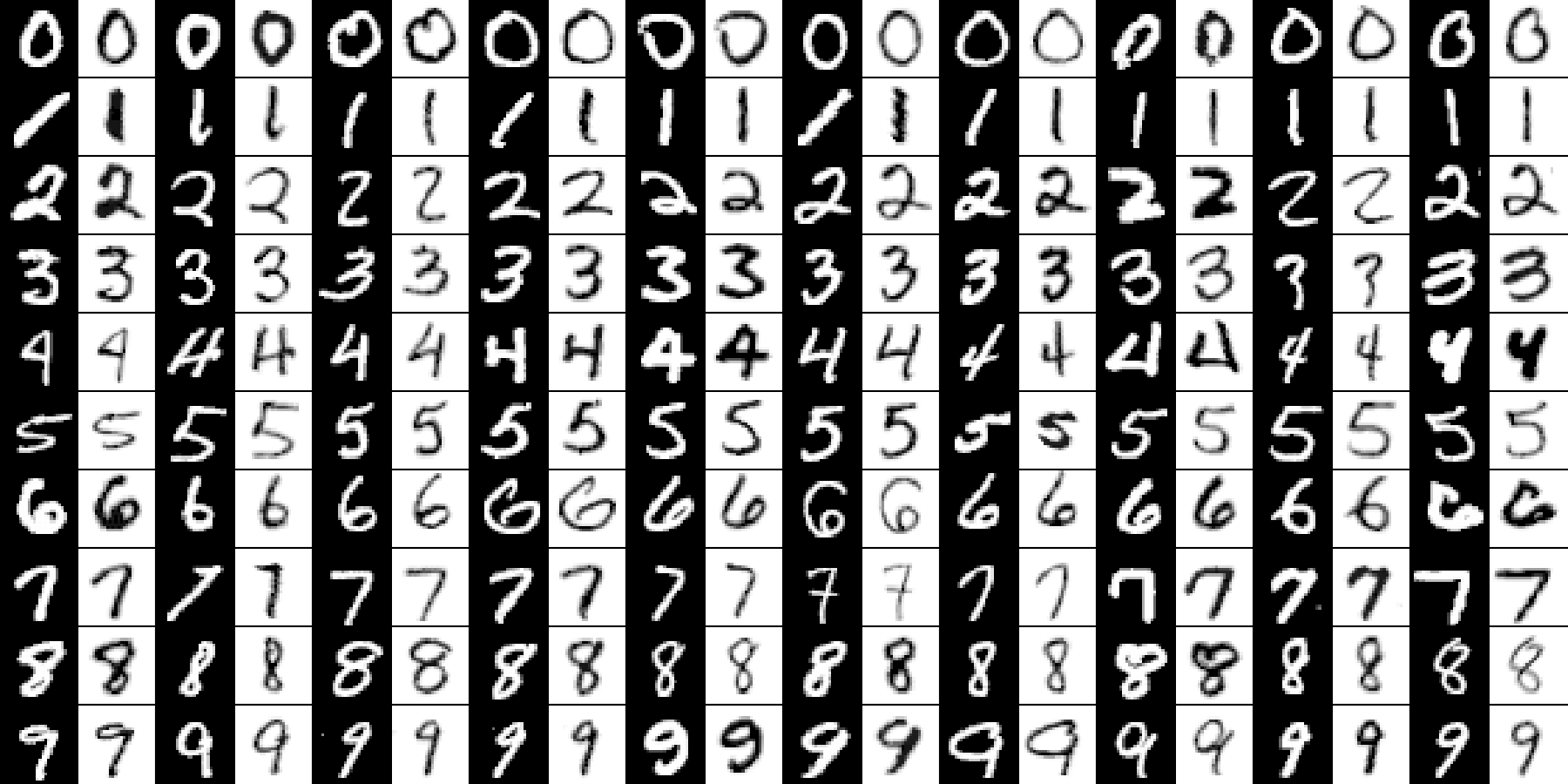## 5.5 Preprocessing of Data

### 5.5.1 Introduction

Do not underestimate the power of appropriate data preprocessing — deep neural networks are not a universal replacement for a data engineer’s hard work!

On top of that, they are not interpretable – those are merely black-boxes.

Among the typical transformations of the input images we can find:

• normalisation of colours (setting brightness, stretching contrast, etc.),
• repositioning of the image (centring),
• deskewing (see below),
• denoising (e.g., by blurring).

Another frequently applied technique concerns an expansion of the training data — we can add “artificially contaminated” images to the training set (e.g., slightly rotated digits) so as to be more ready to whatever will be provided in the test test.

### 5.5.2 Image Deskewing

Deskewing of images (“straightening” of the digits) is amongst the most typical transformations that can be applied on MNIST.

Unfortunately, we don’t have the necessary mathematical background to discuss this operation in very detail.

Luckily, we can apply it on each image anyway.

See the GitHub repository at https://github.com/gagolews/Playground.R for an example notebook and the deskew.R script.

# See https://github.com/gagolews/Playground.R
source("~/R/Playground.R/deskew.R")
# new_image <- deskew(old_image)In each pair, the left image (black background) is the original one, and the right image (palette inverted for purely dramatic effects) is its deskewed version.

Deskew everything:

Z_train <- X_train
for (i in 1:dim(Z_train)) {
Z_train[i,,] <- deskew(Z_train[i,,])
}
Z_train2 <- matrix(Z_train, ncol=28*28)

Z_test <- X_test
for (i in 1:dim(Z_test)) {
Z_test[i,,] <- deskew(Z_test[i,,])
}
Z_test2 <- matrix(Z_test, ncol=28*28)

Multinomial logistic regression model (1-layer NN):

model <- keras_model_sequential()
layer_dense(model, units=10, activation='softmax')
compile(model, optimizer='sgd',
loss='categorical_crossentropy')
fit(model, Z_train2, Y_train2, epochs=5)

Y_pred2 <- predict(model, Z_test2)
Y_pred <- apply(Y_pred2, 1, which.max)-1 # 1..10 -> 0..9
mean(Y_test == Y_pred) # accuracy on the test set
##  0.9433

Performance metrics for each digit separately:

i Acc Prec Rec F TN FN FP TP
0 0.9933 0.9471107 0.9867347 0.9665167 8966 13 54 967
1 0.9951 0.9763158 0.9806167 0.9784615 8838 22 27 1113
2 0.9864 0.9516129 0.9147287 0.9328063 8920 88 48 944
3 0.9906 0.9598394 0.9465347 0.9531406 8950 54 40 956
4 0.9873 0.9313824 0.9399185 0.9356310 8950 59 68 923
5 0.9886 0.9410431 0.9304933 0.9357384 9056 62 52 830
6 0.9903 0.9536354 0.9446764 0.9491348 8998 53 44 905
7 0.9881 0.9595551 0.9231518 0.9410015 8932 79 40 949
8 0.9839 0.9012833 0.9373717 0.9189733 8926 61 100 913
9 0.9830 0.9084713 0.9246779 0.9165029 8897 76 94 933